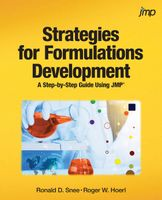Our World Statistics Day conversations have been a great reminder of how much statistics can inform our lives. Do you have an example of how statistics has made a difference in your life? Share your story with the Community!
Choose Language Hide Translation Bar
Formulations involving both mixture and process variables

Ronald Snee and Roger Hoerl have written a book called Strategies for Formulations Development. It is intended to help scientists and engineers be successful in creating formulations quickly and efficiently.

The following tip is from this new book, which focuses on providing the essential information needed to successfully conduct formulation studies in the chemical, biotech and pharmaceutical industries:Although most journal articles present mixture experiments and models that only involve the formulation components, most real applications also involve process variables, such as temperature, pressure, flow rate and so on. How should we modify our experimental and modeling strategies in this case? A key consideration is whether the formulation components and process variables interact. If there is no interaction, then an additive model, fitting the mixture and process effects independently, can be used:

c(x,z) = f(x) + g(z), where 1

f(x) is the mixture model, and g(z) is the process variable model. Independent designs could also be used. However, in our experience, there is typically interaction between mixture and process variables. What should we do in this case? Such interaction is typically modeled by replacing the additive model in Equation 1 with a multiplicative model:

c(x,z) = f(x)*g(z) 2

Note that this multiplicative model is actually non-linear in the parameters. Most authors, including Cornell (2002), therefore suggest multiplying out the individual terms in f(x) and g(z) from Equation 2, creating a linear hybrid model. However, this tends to be a large model, since the number of terms in linearized version of c(x,z) will be the number in f(x) times the number in g(z). In Cornell’s (2002) famous fish patty experiment, there were three mixture variables (7 terms) and three process variables (8 terms), but the linearized c(x,z) had 7*8 = 56 terms, requiring a 56-run hybrid design.

Recent research by Snee et al. (2016) has shown that by considering hybrid models that are non-linear in the parameters, the number of terms required, and therefore the size of designs required, can be significantly reduced, often on the order of 50%. For example, if we fit equation 2 directly as a non-linear model, then the number of terms to estimate is the number in f(x) plus the number in g(z); 7 + 8 = 15 in the fish patty case. Snee et al. (2016) showed using real data that this approach can often provide reasonable models, allowing use of much smaller fractional hybrid designs. We therefore recommended an overall sequential strategy involving initial use of fractional designs and non-linear models, but with the option of moving to linearized models if necessary.